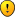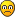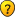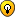## A basketball riddle

Anything goes, but keep it seemly...

### A basketball riddle

Hud will like this one...Riddler wrote:Shaquillina O'Neal, daughter of a famous NBA star, joins the WNBA in the 2018 season... Unlike her dad, she is a very good free throw shooter, and after the first 5 games her free throw percentage stands at .900 (90%)...

To even improve the percentage, her dad decides to give her some "valuable advices" on the shooting movements... After that, her percentage drops dramatically, and stands at .650 (65%) after the first 25 games...

Determined to take matters into her own hand, Shaquillina refuses to listen to her dad anymore, and reverts back to her original movements... At the end of the regular season, her free throw percentage stands at .850 (85%)...

Here are 2 questions:

1. Must there be a particular instance from the 6th game to the 25th game that her free throw percentage stands at exactly .800 (80%)?

2. Must there be such an instance from the 26th game to the last game of the regular season?
udosuk

Posts: 2698
Joined: 17 July 2005

As a wild guess, since I know nothing about basket ball, but going by the phrasing of the questionsudosuk wrote:1. Must there be a particular instance from the 6th game to the 25th game that her free throw percentage stands at exactly .800 (80%)?

No.

udosuk wrote:2. Must there be such an instance from the 26th game to the last game of the regular season?

Yes.

I'll leave the proof to others.

MCC
MCC

Posts: 1275
Joined: 08 June 2005

### Re: A basketball riddle

Riddler wrote:"...and after the first 5 games her free throw percentage stands at .900 (90%)...
"...her dad decides to give her some "valuable advices" on the shooting movements... After that, her percentage drops dramatically, and stands at .650 (65%) after the first 25 games..."

Firstly, my apologies for this joke udosuk but if Shaquillina is annoyed with her dad and gets caught swinging from the basketball net does she get "suspended" automatically.Now for some serious thinking. In trying to work this out does the "first 25 games" include the "the first five games"?

Cec
Cec

Posts: 1039
Joined: 16 June 2005

### Re: A basketball riddle

Cec wrote:Now for some serious thinking. In trying to work this out does the "first 25 games" include the "the first five games"?

Yes...

Sorry about the confusion... By "free throw percentage" I mean the total number of free throws made divided by the total number of free throws taken, cumulative from game 1 to the current time... So the .900 percentage is from game 1 to game 5, .650 is from game 1 to game 25, and .850 is from game 1 to the last game of the regular season, i.e. the whole regular season (where the highest percentage achiever would be awarded the "Free Throwing Queen" award)...

I don't know how much does the phrasing of my questions give away the answer but I'll refrain from commenting on MCC's answers until someone else gives a more elaborated attempt...
udosuk

Posts: 2698
Joined: 17 July 2005

### Re: A basketball riddle

udosuk wrote:Q1. Must there be a particular instance from the 6th game to the 25th game that her free throw percentage stands at exactly .800 (80%)?

For Q1. For the first five games assume Shaquillina "nets" 45 of her 50 throws which would be a 90% success rate. For the next five games assume Shaquillina "nets" 35 of 50 which means for the first ten games she would have "netted" 80 of her 100 throws which gives her an 80% success rate. As this is a particular instance from the 6th to the 25th game meaning it occured at the end of game 10 then is this a correct answer for Q1?

Haven't studied Q2 properly yet.

Cec
Cec

Posts: 1039
Joined: 16 June 2005

Cec, you've shown that it is possible Shaquillina's FT% stands at .800 at one instance from the 6th to the 25th game. That's half the step you need to do for Q1. So congratulations.What now you need to do is to show that if that is a must happening case or not, i.e. if Shaquillina must have such an instance from the 6th to the 25th game.

Basically, for both questions there are 3 scenarios:
(a) Impossible: Shaquillina cannot have any instance where her FT% stands at .800...
(b) Possible but not always: Shaquillina could have such an instance, but it's also possible she does not have one...
(c) Always/Must: No matter what Shaquilla must have an instance that her FT% stands at .800... (This is the hardest to prove...)

So, you've successfully eliminated scenario (a) for Q1. Now you need to eliminate one of (b) or (c) for Q1 to get the correct answer.

Similarly, you have to eliminate 2 scenarios for Q2 (or prove that exactly one of them must be true)...

Carry on!udosuk

Posts: 2698
Joined: 17 July 2005

udosuk wrote:Basically, for both questions there are 3 scenarios:
(a) Impossible: Shaquillina cannot have any instance where her FT% stands at .800...
(b) Possible but not always: Shaquillina could have such an instance, but it's also possible she does not have one...
(c) .............

The answer is (b). The following sequence transitions from 90.0% to 65.0% hitting 80.0% exactly along the way.
Code: Select all
` 9 of 10 = 0.9000 9 of 11 = 0.818210 of 12 = 0.833310 of 13 = 0.769211 of 14 = 0.785712 of 15 = 0.800012 of 16 = 0.750012 of 17 = 0.705913 of 18 = 0.722213 of 19 = 0.684213 of 20 = 0.6500`

The following sequence makes the same transition "stepping over" the 80% number.
Code: Select all
` 9 of 10 = 0.9000 9 of 11 = 0.8182 9 of 12 = 0.750010 of 13 = 0.769210 of 14 = 0.714311 of 15 = 0.733311 of 16 = 0.687512 of 17 = 0.705912 of 18 = 0.666713 of 19 = 0.684213 of 20 = 0.6500`

To keep the examples simple, Shaquillina didn't get to shoot many free throws.ronk
2012 Supporter

Posts: 4764
Joined: 02 November 2005
Location: Southeastern USA

Possible Scenario:
Code: Select all
` 9 of 10 = 0.9000 12 of 15 = 0.800016 of 20 = 0.800020 of 25 = 0.800024 of 30 = 0.800026 of 40 = 0.650056 of 70 = 0.800060 of 75 = 0.800085 of 100= 0.8500`

Now as you can see from the possibilities of .8 in the 1st 100 throws
Code: Select all
` 4 of 5  = 0.8000 8 of 10 = 0.800012 of 15 = 0.800016 of 20 = 0.800020 of 25 = 0.800024 of 30 = 0.800028 of 35 = 0.800032 of 40 = 0.800036 of 45 = 0.800040 of 50 = 0.800044 of 55 = 0.800048 of 60 = 0.800052 of 65 = 0.800056 of 70 = 0.800060 of 75 = 0.800064 of 80 = 0.800068 of 85 = 0.800072 of 90 = 0.800076 of 95 = 0.800080 of 100= 0.8000`

The differences are increments of 1, to me that means that you must hit .8000 exactly as you climb from anything below .8000 to anything above .8000

tarektarek

Posts: 2976
Joined: 05 January 2006

Great analyses ronk & tarek!Okay, so it's settled that Q1's answer is (b) and Q2's answer is (b) or (c). Tarek's explanation that (c) must be correct is not clear enough to me...Perhaps someone could post a more elegant reasoning?

PS: In a whole season it's not unusual for players to shoot 500-600 FTs...
udosuk

Posts: 2698
Joined: 17 July 2005

udosuk wrote:PS: In a whole season it's not unusual for players to shoot 500-600 FTs...

Rather than pick numbers, we can generalize. Let 'M' be free throws Made ... and 'A' be free throws Attempted.

For Q1, when a free throw is missed ...

M/A >= 4/5 >= M/(A+1)

... and one of the equalities will occur when M is evenly divisible by 4.

For Q2, when a free throw is made ...

M/A <= 4/5 <= (M+1)/(A+1)

... but so far I'm unable to draw a conclusion from this. MCC [edit: and tarek] evidently believe one of the equalities must occur.
Last edited by ronk on Wed Aug 09, 2006 9:30 am, edited 2 times in total.
ronk
2012 Supporter

Posts: 4764
Joined: 02 November 2005
Location: Southeastern USA

ronk, what should I say... You're a half step from the destination now...PS: The one you refered to should be tarek... MCC hasn't shared his wisdom on this one yet...udosuk

Posts: 2698
Joined: 17 July 2005

[edit: Incorrect conclusion for Q2 deleted. This "half-step" is a biggie for me.]
Last edited by ronk on Wed Aug 09, 2006 11:36 am, edited 4 times in total.
ronk
2012 Supporter

Posts: 4764
Joined: 02 November 2005
Location: Southeastern USA

I'm not that good at maths, I'll use some of ronk's terminology here, I'm sure a more elegant explanation exists...

M = Free throws scored
A = Free throws attempted
FT percentage= M/A

A free throw = 1
M+1 => A+1 the reverse is not true....

.8000 percent can be expressed in its simplest form in basketball terms as M=4 & A=5 (you cant express that in NBA with smaller numbers)...

Note that A-M=1 (which is the equivelant of 1 free throw)
the next .8000 would be the 8/10 (M=8 & A=10) ...here A-M=2

Note that (A2-M2)-(A1-M1)=1

This goes on for any expression 0f .8000 that follows (the same rationale would decribe an ratio expressed by x/x+1, examples include 1/2=.5 2/3=.6667 4/5=.8 5/6=.8333 6/7=.8571 7/8=.875 8/9=.8889)

at .65 A0-M0 would be an integer
at .90 A1-M1 is also an integer

(from what I mentioned earlier if A1 is around M0 then this is the fastest approach to .9000)

Anyway as all integers are multiples of 1........ climbing from .65 to .8 should always produce an integer A-M that is why it will she will pass through 2/3=.6667 4/5=.8 5/6=.8333 6/7=.8571 7/8=.875 8/9=.8889 & that is why you see those ratios frequently in NBA

tarektarek

Posts: 2976
Joined: 05 January 2006

As I said in my post, I was going by "the phrasing of the questions", in fact, I originly had the answers the other way round, yes to 1 and no to 2.

I won't use probabilities as I will probably mess it up, but in general -

Let's say the question was about the average height of trees in a forest.

You know from a booklet you been given that the average height is 80ft.

If you took a random sample of 50 trees and measured the average, what is the probability you'll come up with 80ft as the averageCompared that to a random sample of 1000 treesThe more you have in a sample the more accurate you can be.

So my thinking (probably totally up the creek) was that the more throws you had, the closer to an actual figure (here 0.80%)you could get.

MCC
MCC

Posts: 1275
Joined: 08 June 2005

Before MCC confuse us more with his forestry analogy (an interesting topic nonetheless), which is not that relevant to this problem, perhaps I'd better demonstrate it myself (as I think most have essentially "grabbed" the main concept here)...

Q1:
ronk has already given 2 separate examples to show that Shaquillina could or could not has her FT% touching the .800 mark. So the answer is (b), neither impossible nor a must.

For it to happen, either M/A=4/5 or M/(A+1)=4/5, i.e. 5M=4A or 4A+4, which is possible only if A or A+1 is a multiple of 5 and M is a multiple of 4.

For it to not happen, M/A > 4/5 > M/(A+1), i.e. 4A < 5M < 4A+4, so there must be a multiple of 5 among 4A+1, 4A+2, 4A+3. For example, A=7, then 4A+2=30 and M could be 6, or A=38, then 4A+3=155 and M could be 31. (Check: 6/7 > 4/5 > 6/8, 31/38 > 4/5 > 31/39...)Q2:
The answer is (c), i.e. Shaquillina's FT% must at one stage be exactly .800. Suppose the contrary is true, i.e. she "jumped" from below .800 to above .800 without stepping on the line, then we must have:

M/A < 4/5 < (M+1)/(A+1)

Which leads to the following 2 inequalities:

5M < 4A so 4A-5M > 0
4(A+1) < 5(M+1) so 4A-5M < 5-4 so 4A-5M < 1

Since both A and M are integers, 4A-5M must be an integer. There is no integer existing between 0 and 1, so we reach a contradiction. Therefore Shaquillina's FT% could not have "jumped" from .800- to .800+ without hitting .800 at least once...

Note that there is 1 exception to the above reasoning... Suppose A=0 and M=0 (i.e. she hasn't shot a FT at all), from basketball convention her FT% is defined as 0% (although mathematically speaking 0/0 is not a valid fraction). If she makes her 1st ever FT, then her new FT% is (0+1)/(0+1)=1=100%, and she has "jumped" over the .800 line...

However, for this particular problem we know it is not the case (she was trying to "jump" from .650 to .850), so this reasoning stands and we can be sure of the answer...Therefore both tarek and MCC has got the correct answers to both (though not with the elegant proof I had in mind)... I'm sure ronk and others could more or less guess it anyway...udosuk

Posts: 2698
Joined: 17 July 2005

Next

Return to Coffee bar